• ## VBA之for循环使用

千次阅读 2020-03-25 22:06:26
有这样的工作表 现在想把E列里,男对应的F称呼列...这里就用到了for循环和if Sub for_if_test() For i = 26 To 2 Step -1 ’ 处理性别 If Range(“e” & i ) = "男) Then Range(“f” & i) = “先生” Else...
有这样的工作表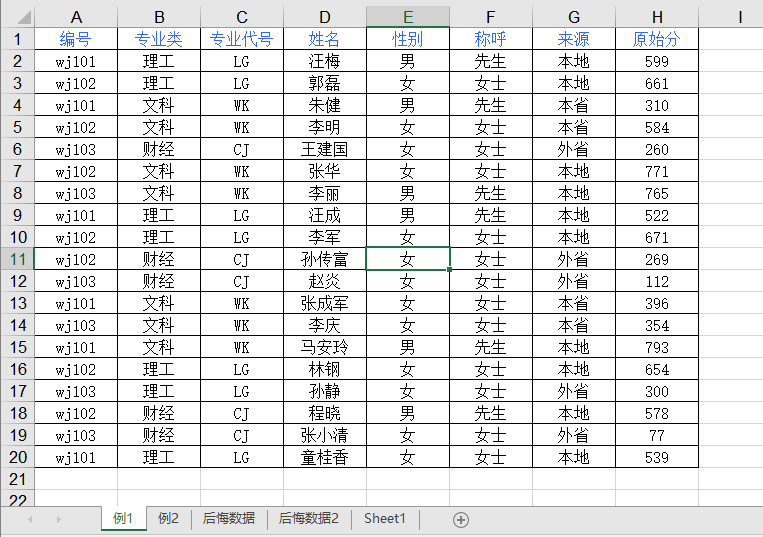现在想把E列里,男对应的F称呼列改为先生,女改为女士 B列的理工对应的C列改为LG,文科改为WK,财经改为CJ,如何去实现?
这里就用到了for循环和if Sub for_if_test() For i = 26 To 2 Step -1 ’ 处理性别 If Range(“e” & i ) = "男) Then Range(“f” & i) = “先生” Else Range(“f” & i) = “女士” '处理专业代号 If Range(“b” & i) = “理工” Then Range(“c” & i) = “LG” ElseIf Range(“b” & i) = “文科” Then Range(“c” & i) = “WK” Else Range(“c” & i) = “CJ” End If
If Range("d" & i) = "" Then
Range("D" & i).Select
Selection.EntireRow.Delete
End If

Next
End Sub
展开全文• 1 for循环的自变量i会停留在i+1 for循环结束后，如果继续查看i 会发现i 会停留在i+1, 但不会停留在i+1之外更大的数字 Sub test14() For i = 1 To 10 '循环结束会挪...for循环中，如果自变量被改变了，自变量会接...

1 for循环的自变量i会停留在i+1
for循环结束后，如果继续查看i会发现i 会停留在i+1, 但不会停留在i+1之外更大的数字
Sub test14()

For i = 1 To 10  '循环结束会挪到下一个i，且停住！
Debug.Print i
Next
Debug.Print i
Debug.Print i
Debug.Print i

End Sub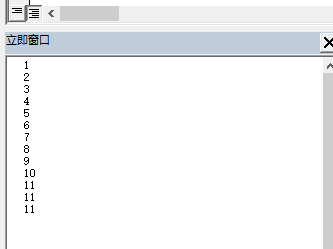2 for循环的自变量i会一直往前走
for循环中，如果自变量被改变了，自变量会接着当前的i值继续往前走下面时个错误的 for 循环，自己写错了，将错就错看看 i的变化

2.1 下面这个是  for 循环过程中，加速i的增长，都是根据实际的i循环
Sub test13()

For i = 1 To 10  '错误的 for 循环，看出i是一个个拿出，已拥有了会到下一个。
Debug.Print "前i=" & i
i = i + 1
Debug.Print "后i=" & i
Next

End Sub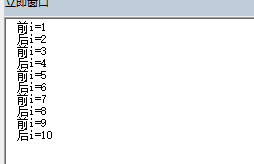2.2 下面这个是for 循环过程中，减慢i的增长，确实都有效
Sub test15()

For i = 1 To 20 Step 2 '错误的 for 循环，看出i是一个个拿出，已拥有了会到下一个。
Debug.Print "前i=" & i
i = i - 1
Debug.Print "后i=" & i
Next

End Sub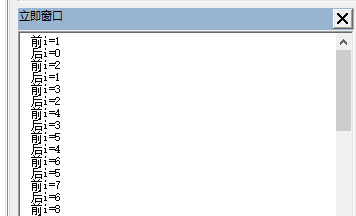展开全文• Option Explicit ... For i = 1 To 100 Step 2 a = a + 1 Range("I" & a).Value = i Next i End Sub Application.Cells Sub 输入3的倍数() Dim i As Byte, xrow As Byte For i = 3 To 100 Step ..

Option Explicit
Sub 输入奇数()
Dim i As Byte, a As Byte

For i = 1 To 100 Step 2
a = a + 1
Range("I" & a).Value = i

Next i
End Sub
Application.Cells

Sub 输入3的倍数()
Dim i As Byte, xrow As Byte
For i = 3 To 100 Step 3
xrow = xrow + 1
Cells(xrow, "J").Value = i
Next i
End Sub

Sub 输入3的倍数2()
Dim i%, xrow%
xrow = 1
For i = 1 To 100 Step 1

If i Mod 3 = 0 Then
Cells(xrow, "H").Value = i
xrow = xrow + 1 '这各xrow变量必须放在if循环里，否则不符合的条件也会增加1，最后导致隔3行才填入1个数据。
End If

Next i

End Sub

Sub 提取工资表名称()
Dim sht As Worksheet, i As Integer '将sht声明为worksheet变量集合
i = 1
For Each sht In Worksheets      'worksheets为worksheet中集合体
Cells(i, "k").Value = sht.Name 'sht.name是worksheet变量里的集合名称
i = i + 1
Next sht
End Sub

Sub 输入100()
Dim arr(1 To 100) As Variant, i%
i = 1
For Each arr(1) In arr
arr(i) = i
Range("L" & i).Value = i
i = i + 1
Next arr

End Sub

Sub 输入100B()
Dim c As Range, i%
i = 1
For Each c In Range("M1:M100")
c.Value = i
i = i + 1
Next
End Sub



展开全文• for循环是一种重复控制结构，它允许开发人员有效地...以下是VBA中for循环的语法。 For counter = start To end [Step stepcount] [statement 1] [statement 2] .... [statement n] [Exit For] [sta...

for循环是一种重复控制结构，它允许开发人员有效地编写需要执行特定次数的循环。
语法
以下是VBA中for循环的语法。

For counter = start To end [Step stepcount]
[statement 1]
[statement 2]
....
[statement n]
[Exit For]
[statement 11]
[statement 22]
....
[statement n]
Next

流程图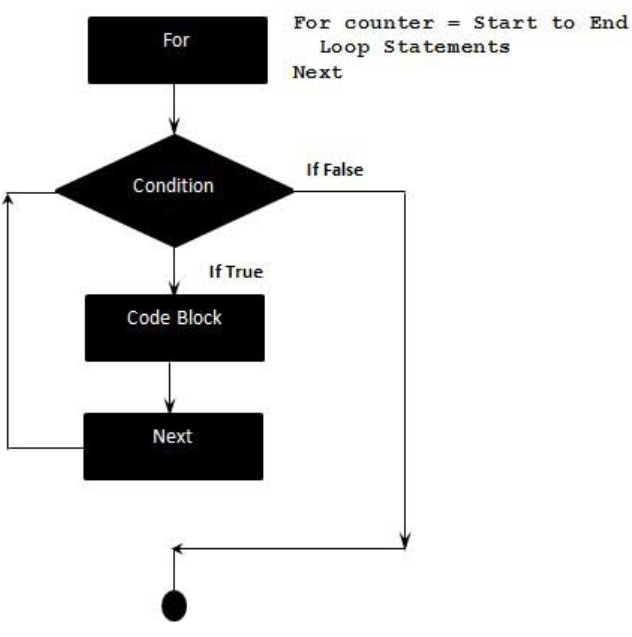以下是For循环中的控制流程 -
For步骤先执行。这一步允许您初始化任何循环控制变量，并递增步进计数器变量。其次，评估条件。 如果评估结果为：True，则循环体被执行。 如果为False，则循环体不会执行，并且控制流将跳转到紧跟在For循环之后的下一个语句。在执行For循环体之后，控制流将跳转到下一个语句。 这个语句更新任何循环控制变量。 它根据步计数器值进行更新。现在条件再次评估。 如果条件为：True，则循环执行并且该过程重复自身(循环体，然后递增步，然后再次条件)。 条件变为False后，For循环终止。
示例
添加一个模块，并添加以下函数代码 -

Private Sub Constant_demo_Click()
Dim a As Integer
a = 10

For i = 0 To a Step 2
MsgBox ("The value is i is : " & i)
Next
End Sub

当上面的代码被编译并执行时，会产生类似以下结果。

The value is i is : 0

The value is i is : 2

The value is i is : 4

The value is i is : 6

The value is i is : 8

The value is i is : 10

转载于:https://www.cnblogs.com/sunyllove/p/11348201.html
展开全文• 数组的应用: 代码1: Sub test() Dim i, j, k, l As Integer ...For i = 2 To 80 For j = 2 To 80 For k = 2 To 80 For l = 2 To 80 If Range("a" & i) + Range("a" & j) + Range("a" & k) ...
• ## VBAFor Each循环

千次阅读 2019-05-20 09:40:52
VBA For Each循环 ...For Each循环与For循环类似; 然而，For Each循环是为数组或组的每个元素执行的。 因此，这种类型的循环中将不存在步计数器。 它主要用于数组或在文件系统对象的上下文使用，以...
• 2、生成工资条 代码如下： Sub gzt() Dim i As Integer Rows("1:1").Select For i = 1 To 10 Selection.Copy ActiveCell.Offset(2, 0).Rows("1:1").EntireRow.Select Selection.Insert Shift:=xlDown Next End Sub ...
• 那么通过如下代码，看一下在for循环中，不同的字符串拼接方式，到底哪种效率最高?  package com.test.stringjoin; import org.apache.commons.lang3.StringUtils; public class Test { public static void main...
• 跳出for循环大概三种层次（1）跳出本次for循环，进行本层的下一次循环（2）跳出本层for循环，执行for语句之外的其他语句（3）跳出整个嵌套循环，执行嵌套循环以外的语句本菜写的小例子如下：Sub try() Dim i As ...
• VBA当中，同样和编程语言一样，有着for循环语句。其语法为如下：For<计数器=开始数>To<结束数>[step步长][指令][ExitFor][指令]Next[计数器]从开始到结束，反复执行For和Next之间的指令块，除非遇到...
• 当你知道你需要重复运行多少次某段语句时，可以使用For…Next语句。它的语法如下： For 计数器 = 开始 To 结束 [步长] 语句1 语句2 语句N Next [计数器] 括号里面的代码是可选的。计数器是个储存反复次数的数字型...excel
• 本章主要内容：Excel中宏的使用，手工书写VBA代码，使用For循环，小结及练习。excel
• Excel中宏的使用 录制宏，用于表格完全一样的模式 相对引用宏的录制操作： 具体如下： ... ...使用for循环-解决重复动作 当我想把上面改颜色的动作重复50次时，要一直手动点宏-执行，显然是不合理的..excel
• for循环 Sub gzd() Range("A1:L1").Select Dim i As Integer For i = 1 To 10 ActiveCell.Range("A1:L1").Select Selection.Copy ActiveCell.Offset(2, 0).Rows("1:1").EntireRow.Select Selection.Insert ...程序人生
• ## VBA中的10种循环语句

万次阅读 多人点赞 2019-04-17 12:00:31
VBA中的10种循环语句 1、For – Next '循环数组 ArraySum = 0 For i = 1 To 10 ArraySum = ArraySum + MyArray(i) Next i 2、For Each – Next '循环单元格集合 SelectionSum = 0 For Each cell In Selection ...
• 在单元格区域对象中循环For Each c In Range("$A$2:$A$" & Cells(Rows.count, "A").End(xlUp).row ‘do something Next c 基于上述代码运行后，你会发现，少量数据处理上还行，但大量数据之后，电脑卡慢，...
• 声明：笔记来源于我要自学网-《Excel VBA基础教程》-授课讲师：曾贤志 当需要处理集合成员时，一般会用 For Each...Next,实际上就是处理对象 实例应用：判断是否等于“A1”是就填充底色为红色 Sub foreachnext...
• 代码比较简单，只是在替换的基础上增加了最基础的For循环，有效节省了批量替换文本的时间。 废话不多说，直接上代码， Ori = Array("a","b","c") '被替换文本 Rep = Array("a&...WORD
• for循环略慢foreach 对于链表来说 for循环比foreach慢很多。因为for循环每次获取链表节点都要重头开始查找，而foreach是采用迭代方式，查找节点只需遍历一次，节省了很多时间。 总结 在循环操作不涉及插入、...
• '同一个存在于某固定行数的For循环体系如果没跑完不能回到之前并继续进行同一个(存在于某固定行数)的循环, 否则报错 '该数组被固定或暂时锁定, 英文: This array is fixed or temporarily locked. '如果是到了另一个...
• VBA退出循环用Exit语句，在循环中使用Exit语句相当于C/C++的break语句，而在函数中使用Exit语句则相当于C/C++的return语句。  一、退出循环。exit for退出for循环，exit do用于退出do 或 do while循环： '获取非...
• Excel：VBA 数据分析 写一个for循环的单元格判断程序（四 还是那张测试表。 本文要实现的逻辑是：在 F列 实现对 E列 的判断并显示结果。当E列 的单元格 分数 大于 90 分时，则 F列 应该显示判断结果为优秀；当 E列 ...excel 数据分析
• Sub 多张工作表成绩汇总() Dim Z, H2, B 'Z总分；... For B = 1 To Worksheets.Count 'B代表工作表，每一张工作表循环一次。 Set C1 = Worksheets(B) Z = 0 '在第一次执行前总分为0 For H2 = 2 To 11
• For Each 循环 第一块内容：For Each循环 Sub test1() Dim ge As Range'把变量ge定义为Range（rng）类型，单元格变量/区域变量 For Each ge In Range("A1:A10")'在单元格区域A1：A10范围内，循环查找单元格 ge = 1...excel
• 1 问题：什么时候可以用 for each 进行循环？ 不需要知道具体的循环变量，上下限。 答案 : 当for each xx in yyy ，只要 yyy 是 对象集合(s) 比如像workbook 这样的可数的对象的集合就可以 可以用for each 的...
• 第一次写代码-For循环 （Excel基础） 1.Excel 宏的使用 2.手工写VBA代码 3.使用for 1.Excel 宏的使用 准备工作：调出开发工具：文件->选项->自定义功能区->开发工具打钩√ 确定。此时Excel上面会出现...
• for循环是一种重复控制结构，它允许开发人员有效地编写需要执行特定次数的循环...